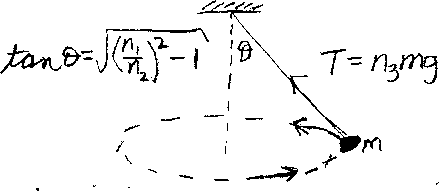Problem B14: Consider the conical pendulum shown above. A bob of mass m is attached to a massless string. The bob moves in a horizontal circle, and the string makes an angle &theta with respect to the vertical. If the tangent of the angle &theta equals tan &theta = √ (n1/n2)2-1 , what is the tension T in the string. If T = n3 mg, what is n3? Note that n1, n2 and n3 are unitless.n1 = n2 = Input n3:
If you are currently in my class, you can record your grade by entering your name and student ID number (without the leading zeros) below and clicking on "record grade".
 First Name = Last Name = ID = Problem: